# Physics 101: Beginner to Intermediate Concepts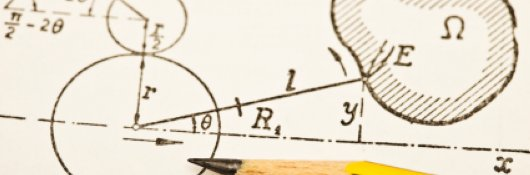\$85.00
no certificate
- OR -
\$110.00
with CEU Certificate*
Start Right Now!
Taking multiple courses? Save with our platinum program.

• 15
Lessons
• 26
Exams &
Assignments
• 1,800
Students
have taken this course
• 24
Hours
average time
• 2.4
CEUs## Course Description

Physics is a foundational science on which many of the concepts of chemistry, geology, biology, and other natural sciences rely. Because physics is a math-intensive subject, it is sometimes intimidating to those who wish to study it.

This course focuses on some of the critical mathematical tools required for studying physics and is geared toward students with a basic knowledge of algebra, geometry, and trigonometry. Starting with an introduction to vectors, the course then begins to develop a set of mathematical tools for studying classical Newtonian mechanics as well as some basic topics in classical electromagnetism. Although the course does not cover calculus, the math that is presented is more than sufficient for analyzing and solving a wide range of problems involving the motion of objects and forces such as gravity, the electric force, and magnetism.

The first half of the course deals with objects in motion, including how to quantify and relate characteristics such as force, velocity, momentum, energy, and power. The second half of the course focuses on waves and electromagnetism, starting with a look at the electric and magnetic forces and their sources, then moving to acoustic and electromagnetic waves, and ending with an overview of geometrical optics. By the end of the course, students should be armed with many of the tools they need to study physics in depth.
• Completely Online
• Self-Paced
• Printable Lessons
• Full HD Video
• 6 Months to Complete
• Start Anytime
• PC & Mac Compatible
• Android & iOS Friendly
• Accredited CEUs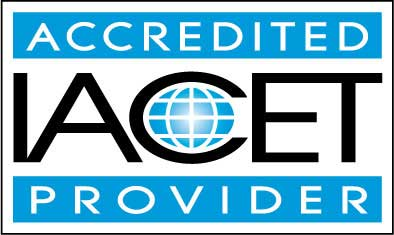## Course Lessons

Average Lesson Rating: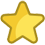#### Lesson 1 - Introduction and Mathematical Foundations

This lesson discusses some simple, but fundamental, mathematical tools that will underlie the principles and methods presented in the remainder of the course. 19 Total Points
•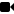Lesson 1 Video
•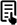Review Article: Understanding Vectors
•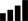Take Survey: Reasons for Taking this Course
•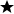Complete: Lesson 1 Assignment
•Complete Exam: Lesson 1 – Introduction and Mathematical Foundations

#### Lesson 2 - Force and Newton's Laws I

In the previous lesson, we introduced the idea of vectors as quantities with magnitude (or length) and direction. We now take a deeper look at the properties of vectors in the context of forces and Newton's first law of motion. 18 Total Points
•Lesson 2 Video
•Complete: Lesson 2 Assignment
•Complete Exam: Lesson 2 - Force and Newton's Laws I

#### Lesson 3 - Force and Newton's Laws II

Building on the material we studied in the previous two lessons, we now apply our understanding of vectors and forces to Newton's second and third laws of motion. 14 Total Points
•Lesson 3 Video
•Complete: Lesson 3 Exercises
•Complete Exam: Lesson 3 – Force and Newton's Laws II

#### Lesson 4 - Uniform Circular Motion

In this lesson, we look at how to apply both vectors and the geometry of circles and triangles to uniform circular motion. Examples of such motion include the orbits of celestial objects, such as planets and stars. 13 Total Points
•Lesson 4 Video
•Complete Exam: Lesson 4 Uniform Circular Motion
•Complete: Lesson 4 Exercises

#### Lesson 5 - Momentum

In this lesson, we define the concept of momentum in the context of physics and use the mathematics of vectors and differences (such as the difference in time, Δt) to derive the law of conservation of linear momentum 18 Total Points
•Lesson 5 Video
•Complete: Lesson 5 Assignment
•Complete Exam: Lesson 5 Momentum

#### Lesson 6 - Work

In this lesson, we turn from momentum to work, which is the amount of energy required to perform a given task (such as moving an object from one point to another). 11 Total Points
•Lesson 6 Video
•Complete: Lesson 6 Exercises
•Complete Exam: Lesson 6 Work

#### Lesson 7 - Work and Kinetic Energy

This lesson takes the concept of work developed in the previous lesson and applies it to the concept of kinetic energy, or energy of motion. 12 Total Points
•Lesson 7 Video
•Complete: Lesson 7 Exercises
•Complete Exam: Lesson 7 Work and Kinetic Energy

#### Lesson 8 - Mechanical Energy and Power

This lesson concludes our look at basic Newtonian mechanics (motion) by considering potential energy and its relationship to kinetic energy through the concept of mechanical energy. 10 Total Points
•Lesson 8 Video
•Complete Exam: Lesson 8 Mechanical Energy and Power

#### Lesson 9 - Electrostatics

The course now turns from an examination of the mechanics of motion resulting from applied forces to an overview of a specific type of force: the electric force. 9 Total Points
•Lesson 9 Video
•Complete Exam: Lesson 9 Electrostatics

#### Lesson 10 - Currents and Magnetism

In this lesson, we turn from stationary charges to charges in motion. Charged particles in motion exert a magnetic force on each other. 18 Total Points
•Lesson 10 Video
•Complete: Lesson 10 Exercises
•Complete Exam: Lesson 10 Currents and Magnetism

#### Lesson 11 - Waves

This lesson considers some of the mathematical aspects of waves that we will need to briefly study sound and light in later lessons. 9 Total Points
•Lesson 11 Video
•Complete Exam: Lesson 11 Waves

#### Lesson 12 - Acoustic Waves

In this lesson, we begin to apply our knowledge of waves gained in the previous lesson to the topic of sound (acoustic waves). 14 Total Points
•Lesson 12 Video
•Review 2 Articles: Acoustics and Vibration Animations; The Speed of Sound
•Complete: Lesson 12 Exercises
•Complete Exam: Lesson 12 Acoustic Waves

#### Lesson 13 - Electromagnetic Waves

In this lesson, we consider electromagnetic waves, including the ways in which they compare and contrast with acoustic waves. 12 Total Points
•Lesson 13 Video
•Complete: Lesson 13 Exercises
•Complete Exam: Lesson 13 Electromagnetic Waves

#### Lesson 14 - Rays: Geometrical Optics I

Although the mathematics associated with waves can be extremely complicated, we can in some cases approximate waves as "rays" (geometrical optics). 10 Total Points
•Lesson 14 Video
•Complete Exam: Lesson 14 Rays: Geometrical Optics I

#### Lesson 15 - Rays: Geometrical Optics II

In this lesson, we briefly look at how geometrical optics can be used to analyze images created by mirrors and lenses. 57 Total Points
•Lesson 15 Video
•Review Article: Ray Diagrams
•Review Video: Geometrical Optics
•Take Poll: What do you think about this course?
•Take Survey: Program Evaluation Follow-up Survey (End of Course)
•Complete Exam: Lesson 15 Rays: Geometrical Optics II
•Complete: The Final Exam
244
Total Course Points

## Learning Outcomes

By successfully completing this course, students will be able to:
• Summarize mathematical foundations of physics.
• Describe force and Newton's Laws.
• Define uniform circular motion.
• Solve problems involving Momentum.
• Solve problems involving Work.
• Solve problems involving Work and Kinetic Energy.
• Solve problems involving Mechanical Energy and Power
• Summarize electrostatics.
• Solve problems involving Currents and Magnetism
• Summarize waves.
• Summarize acoustic waves.
• Summarize electromagnetic waves.
• Describe Geometrical Optics, and
• Demonstrate mastery of lesson content at levels of 70% or higher.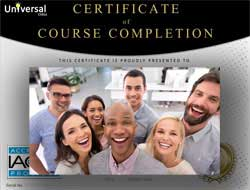• Document Your Lifelong Learning Achievements
• Earn an Official Certificate Documenting Course Hours and CEUs
• Verify Your Certificate with a Unique Serial Number OnlineCourse Title: Physics 101: Beginner to Intermediate Concepts
Course Number: 9770457
Lessons Rating: 4.7 / 5 Stars (489 votes)
Languages: English - United States, Canada and other English speaking countries
Category:
Course Type: General Education (Self-Paced, Online Class)
CEU Value: 2.4 IACET CEUs (Continuing Education Units)
CE Accreditation: Universal Class, Inc. has been accredited as an Authorized Provider by the International Association for Continuing Education and Training (IACET).
Assessment Method: Lesson assignments and review exams
Course Author: Dr. Jeffrey Clark, Ph.D.
Instructor: April Graves
Syllabus: View Syllabus
Duration: Continuous: Enroll anytime!
Course Fee: \$85.00 (no CEU Certification) || with Online CEU Certification: \$110.00

Course Only
One Course
No Certificate / No CEUs
\$85
for 6 months

Billed once
This course only
 Includes certificate X Includes CEUs X Self-paced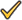Instructor supportTime to complete 6 months No. of courses 1 course
Certificate Course
One Course
Certificate & CEUs
\$110
for 6 months

Billed once
This course only
 Includes certificateIncludes CEUsSelf-pacedInstructor supportTime to complete 6 months No. of courses 1 course
Platinum Yearly
ALL COURSES
Certificates & CEUs
\$189
per year
You save 50%!
Billed once
Includes all 500+ courses
 Includes certificateIncludes CEUsSelf-pacedInstructor supportTime to complete 12 Months No. of courses 500+
Platinum 2 Years
ALL COURSES
Certificates & CEUs
\$299
for 2 years
You save \$427.00!
Billed once
Includes all 500+ courses
 Includes certificateIncludes CEUsSelf-pacedInstructor supportTime to complete 24 Months No. of courses 500+

## Student Testimonials

• "Instructor was very prompt in correcting assignments and exams, with details given of mistakes" -- Colleen M.
• "Everything was great, thank you!...Awesome course, I appreciated the feedback." -- Shayana M.